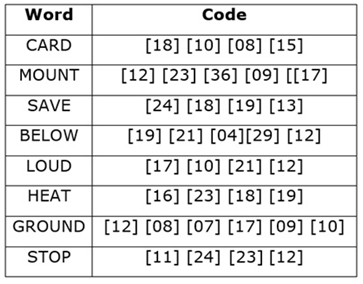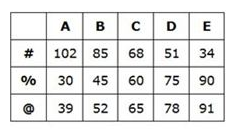# IBPS PO Mains Reasoning (Day-02)

Dear Aspirants, Our IBPS Guide team is providing new series of Reasoning Questions for IBPS PO Mains 2020 so the aspirants can practice it on a daily basis. These questions are framed by our skilled experts after understanding your needs thoroughly. Aspirants can practice these new series questions daily to familiarize with the exact exam pattern and make your preparation effective.

Start Quiz

Matrix based puzzle

Directions (1-4): Study the following information carefully and answer the below questions.

There is a 3*5 matrix that can produce signals which in turn help in the illumination of some bulbs. The row of the matrix is denoted by @, % and # from the bottom to top respectively and the columns are denoted by A, B, C, D and E from left to right respectively.

@ row contains a number which are consecutive multiples of 13, starting from 39 from left to right. % row contains a number which are consecutive multiples of 15, starting from 30 from left to right. # row contains a number which are consecutive multiples of 17 starting from 34 from right to left.

The matrix helps in producing signals which can be either a single string of number X or two line string X and Y. There are four lights P, Q, R and S. Based on the outcome of the string mentioned above one of the light blinks.

a) If the outcome is below 50 then P will blink

b) If the outcome range is 51-200, then Q will blink

c) If the outcome range is 201-270, then R will blink

d) If the outcome is greater than 270 then S will blink

For outcome of the string:

1) If the string has all even numbers then the outcome of the string is obtained by adding all the numbers.

2) If an odd number is followed by an even number then, the one’s place of all the two digit numbers is deleted and 10th place is multiplied to get the outcome.

3) If the string has a prime number, then the 10th place is deleted from each of the two digit numbers and the remaining numbers are multiplied.

4) If no above logic is followed then outcome is an addition of the number.

1) Which of the following bulb will illuminate for the given input

X = @B%A#[email protected]?

E. Cannot be determined

2) Which of the following bulb will illuminate for the given input

X = %[email protected]#E%A?

E. Cannot be determined

3) Which of the following bulb will illuminate for the given input

X = @B#E%E#A?

E. Cannot be determined

4) Which of the following bulb will illuminate for the given input

X = @E#[email protected]%B?

E.Cannot be determined

Coding decoding

Direction (5-6): In a certain code language letters of the English alphabet is coded as shown in the below table. Numeric codes for each letter are not necessarily in the same orderStudy the following information carefully and answer the below questions.5) How “VALUE” can be coded in the same code language?

A.     

B.     

C.     

D.     

E. None of these

6) How “RANDOM” can be coded in the same code language?

A.      

B.      

C.      

D.      

E. None of these

Input output

Directions (7-10): A machine rearrangement given an input line having both words and numbers in a particular set of rules in step by step. The following is an illustration of input and its rearrangement.

Input: strong 4348 change 7321 brand 1025 1302 cause 6341 great 2712 surface

Step I: brand strong 4348 change 7321 1025 cause 1302 6341 great 2712 surface

Step II: brand 8 strong 4348 change 7321 cause 6 6341 great 2712 surface

Step III: brand 8 change 4348 strong 7321 cause 6 great 6341 2712 surface

Step IV: brand 8 change 13 4348 strong cause 6 great 12 6341 surface

Step V: brand 8 change 13 strong 4348 cause 6 great 12 surface 6341

Step VI: brand 8 change 13 strong 19 cause 6 great 12 surface 14

As per rules followed in the given steps, find appropriate step for given Input.

Input: 7234 science 3416 2103 global contest 5943 last occur 3121 glitch 2643

7) Which of the following element is sixth from the left end in step IV?

A.glitch

B.science

C.3416

D.7234

E.None of these

8) Which of the following step represents the following output ‘contest 6 global 14 science 7234 glitch 7 occur 15 last 5943’?

A.There is no such step

B.Step IV

C.Step II

D.Step III

E. Step I

9) How many elements are there between ‘science’ and ‘last’ in step II?

A.Seven

B.Three

C.Five

D.Six

E.None of these

10) What is the sum of third element from the right end in last step and fourth element from the left end in step III?

A.7250

B.7249

C.21

D.35

E.None of these

Directions (1-5):

From the given data we can create the following table;X = @B%A#[email protected]

X = 52 30 102 78

Here all the outcome of the string is in even number; So,

X = 52+30+102+78=262

X = %[email protected]#E%A

X = 45 65 34 30

Here in the outcome of the string odd number is followed by an even number; So,

X = 4 x 6 x 3 x 3 = 216

X = @B#E%E#A

X = 52 34 90 102

Here all the outcome of the string is in even number; So,

X = 52 + 34 + 90 + 102 = 278

X = @E#[email protected]%B

X = 91 51 39 45

Here in the outcome of the string there is a prime number; So,

X = 1 x 1 x 9 x 5 = 45

Directions (5-6):

We have:

CARD –>     …………. (i)

MOUNT –>     [ ……. (ii)

SAVE –>     ………… (iii)

BELOW –>     …… (iv)

LOUD –>     ……… (v)

HEAT –>     ……….. (vi)

GROUND –>       …………….. (vii)

STOP –>     ………………….. (viii)

From (i), (iii) & (vi) we can says that:

A –>18

E –> 19

Again, from (i), (ii), (iv) & (v) we can say that:

O –>12

U –> 17

D –>10

L –>21

Similarly, after comparing all the above words, we have final decoded codes as follows:

A –> 18; E –> 19, O –>12; U –>17; D –>10; L –> 21; R –> 08; C –>15; N –>09; T –>23; M –> 36; S –> 24; V –>13; H –>16; G –>07; P –>11.

B/W –> 04/29 (Not necessarily in the same order)

Directions (7-10) :

Logic:

In the given input there are twelve elements, in which the first six elements are arranged in one logic, the same logic which is used for second six elements

First six elements are arranged in which words are arranged in ascending order and the numbers are arranged in ascending order with respect to sum of the digits in a number.

For the second six elements, the same logic is applied as in the first six elements.

Input: 7234 science 3416 2103 global contest 5943 last occur 3121 glitch 2643

Step I: contest 7234 science 3416 2103 global glitch 5943 last occur 3121 2643

Step II: contest 6 7234 science 3416 global glitch 7 5943 last occur 2643

Step III: contest 6 global 7234 science 3416 glitch 7 last 5943 occur 2643

Step IV: contest 6 global 14 7234 science glitch 7 last 15 5943 occur

Step V: contest 6 global 14 science 7234 glitch 7 last 15 occur 5943

Step VI: contest 6 global 14 science 16 glitch 7 last 15 occur 21